Introducing Multiplication - Tales from Outside the Classroom

# Introducing Multiplication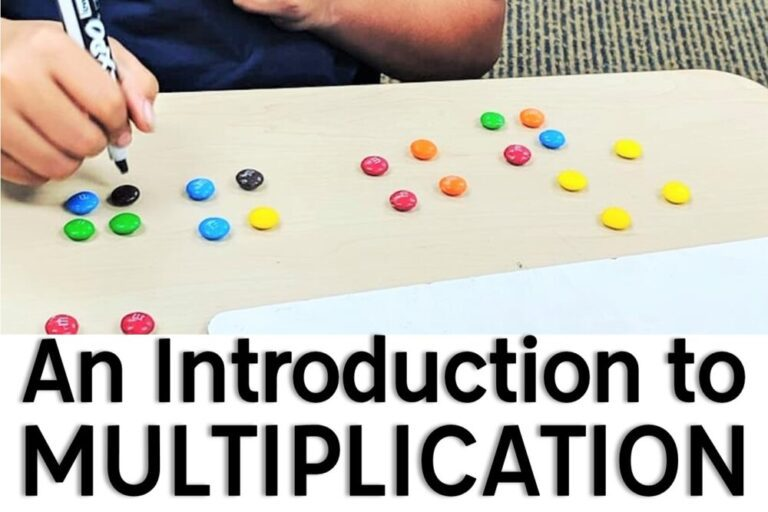Mastery in multiplication and division is built on a solid foundation of addition and subtraction.  Understanding the concepts of multiplication and division rely on a solid mastery of understanding the concepts of whole and total from addition and subtraction.  Unfortunately, we don’t always have the luxury of spending a lot of time reviewing addition and subtraction in third grade before we start multiplication and division.  Because of this, it’s so important for me to connect to addition when introducing multiplication so I can reinforce what students know, or should know, about multiplication to connect the two.

## Introducing Multiplication

Multiplication is finding the total of items organized in an equal grouping arrangement.  Multiplication is always whole unknown, or total unknown.  This is how/why it’s related to addition, which is also total unknown.  It’s crucial that students understand that multiplication is an operation that finds the total number of objects arranged in equal groups.  This becomes key when understanding multi-step and mixed operations word problems.

My favorite math manipulative is food and I use it as often as possible.  To introduce multiplication I pass out a bunch of M&M’s to each student.  I typically don’t count the number as it’s okay if students have different amounts. I then ask them to make “X groups of X” using the M&M’s as manipulatives.  We talk about the necessity for equal groups, as well as the standard formula of

# of groups x # in each group = total

We talk about each equation as “# groups of #”.  We also explore the Commutative Property with our work with the M&M’s, right away, but I introduce the concept of a specific arrangement first.  When we talk about the Commutative Property and explore how it works, we talk about how the two are NOT THE SAME but will always result in the same total.  It’s so important for students to understand that both equations are not the exact same and don’t represent the exact same, but that using the Commutative Property is an important strategy to know to solve an unknown equation.  We use our M&M’s to model both equations to show how they’re similar, but not the same.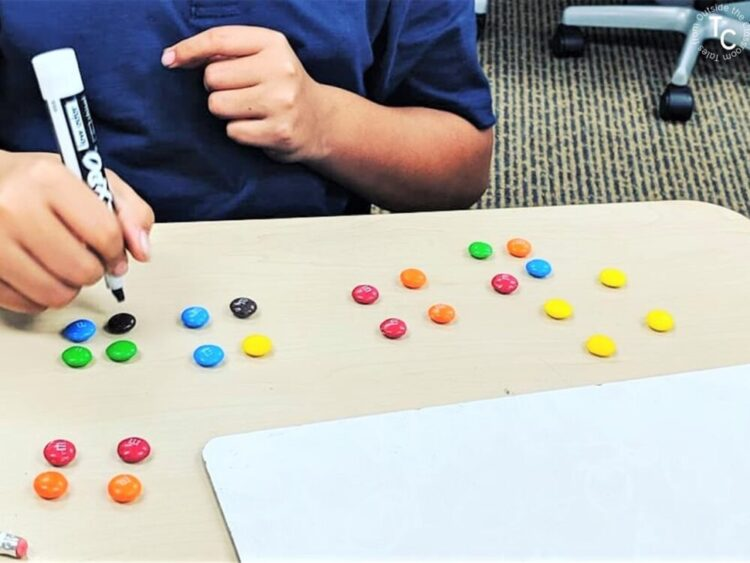After we’ve done a few arrangements with equal groupings, students make arrays using their M&M’s.  Most students are pretty successful with making arrays because they remember arrays from 2nd grade.  As we find new totals to solve equations, we talk about the same phrasings that we did with equal group arrangements, except we say “rows of” instead of “groups of“.  I have found that students understand how both strategies are related when I use the same phrasing.  We also touch on the zero and identity properties of multiplication so students can have a concrete understanding of the properties.  I also use the array and equal groups representations to introduce repeated addition.

Typically the next day I also create a student background knowledge anchor chart on multiplication. Many students have somewhat of an understanding from 2nd grade, as many teachers introduce multiplication facts with their high achieving students. I like to bring students’ knowledge to the forefront and address any misconceptions pretty quickly, and it also gives us a chance to review what we learned already.  We display the anchor and add and refer to it during our unit.  I also use this opportunity to introduce the content vocabulary words of “factor” and “product”.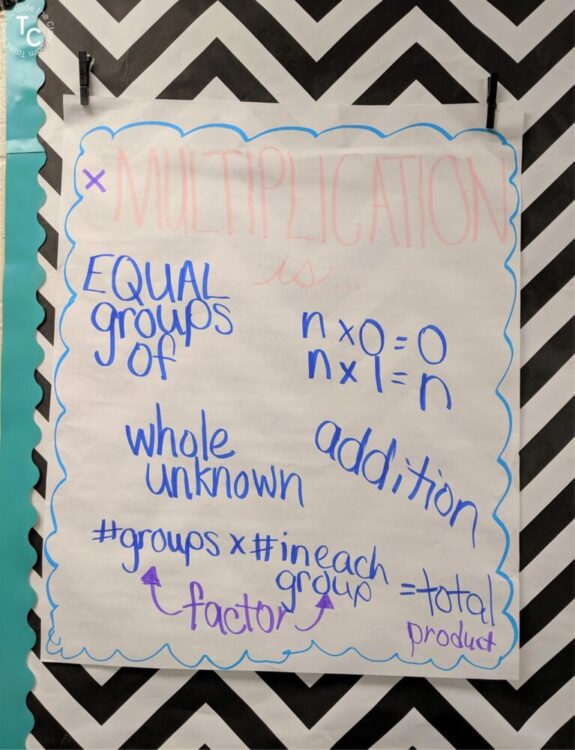## Multiplication Strategies

After the first day, students have a solid concrete understanding of using arrays and equal groups to solve multiplication equations. Our work together as we review focuses on correct strategy use resulting in correct solutions. When I’m introducing multiplication I focus on “x groups of x” and “x rows of x” because I want to ensure students truly understand multiplication. We use my Multiplication and Division Pictures & Arrays Task Cards to practice writing and solving multiplication equations. I usually use these as a Solve the Room- displaying them across the room as students move around to solve them.  Because my class is smaller than the set, I use a few to introduce them together whole group before I have students work on them independently.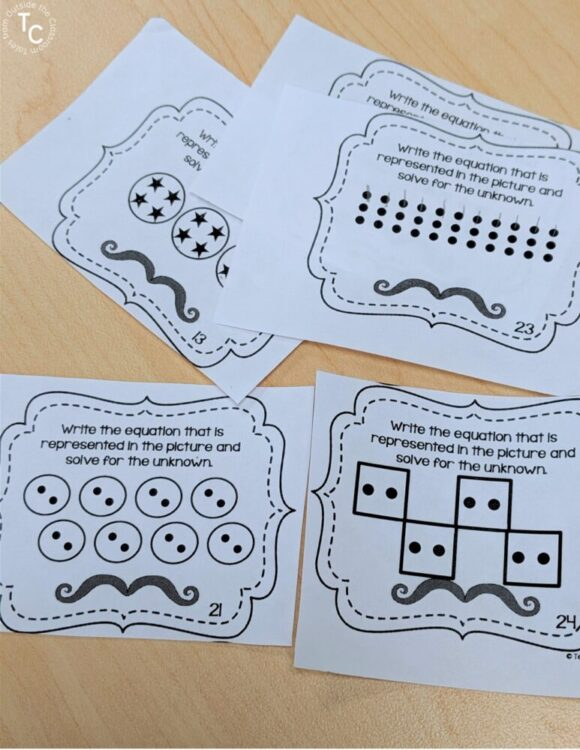After that, my students have a pretty solid understanding of multiplication and using equal groups pictures and arrays. They are ready to move on to other strategies.  I create this anchor chart with students to demonstrate how to use number lines and repeated addition to solve multiplication equations.  We relate the new strategies to equal groups and arrays to show how each of them is related.  I also then introduce my students to skip counting using these tunes from All Things Apple in 2nd. I help students see that skip counting is just repeated addition.  While there are TONS of multiplication facts songs out there, I like to focus on skip counting because students can use it to help them count their array rows and equal groups.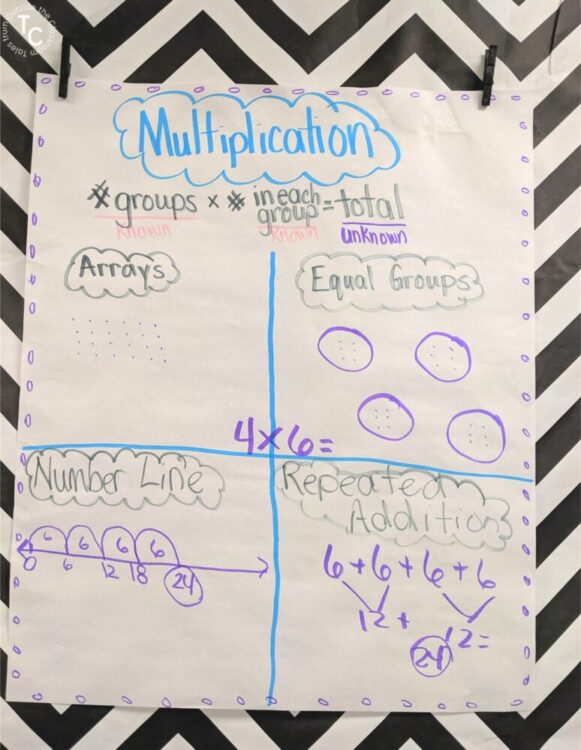The rest of our work is focused on using the multiplication strategies successfully and understanding multiplication scenarios. My work in small groups ensures students are using the strategy of their choice successfully. I also look for any misconceptions. For example, I’ve found kids that just cannot be neat enough to use arrays successfully and I direct them to use equal groups pictures.  Or, others, struggle with OT needs, and arrays and equal groups are too much for them.  I came up with a slightly modified equal groups strategy so students only draw the groups once.  It’s been much more successful with my students who struggle to make their image precisely. You can watch my modified equal groups tutorial on this Instagram highlight.  During our small group work I also force specific strategies so I know students understand each.  Some strategies are dictated in our standards so it’s important that students know and understand the strategies that they don’t choose to always employ.

## Multiplication Practice

After I’ve introduced multiplication and we’ve gone through multiplication strategies, my students practice independently. I have them work through my Introduction to Multiplication Digital Lesson.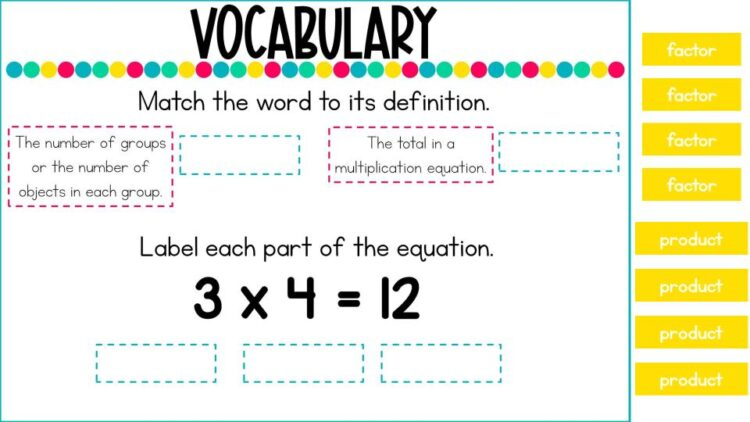The lesson walks students through multiplication from concrete to abstract scenarios. Kids work on building multiplication models, matching multiplication equations to representations, and solve multiplication word problems. The lesson focuses on the multiplication vocabulary of factor and product so students become proficient with academic vocabulary.

Students also complete my digital Multiplication Task Cards included with my Digital Introduction to Multiplication lesson. It gives students independent practice with multiplication, and I’m able to check their progress. The digital task cards are perfect practice for students in math centers when they’re not meeting with me. I’ve also used these digital task cards online during distance learning. Before the week is up, I also check my students’ progress using the included quiz on 3.OA.1.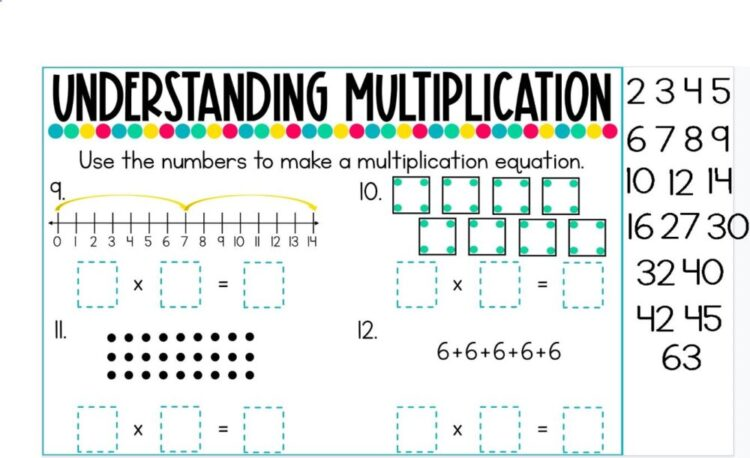I use my Multiplication and Division on Number Lines task cards as part of our whole group and small group instruction.  I rarely see this as a strategy students use, partially due to them not using number lines as a strategy for other math skills.  Building understanding of multiplication on a number line helps build a concrete understanding of multiplication, but also takes a lot of practice.  We use these same task cards later when introducing division, and when we’re working with multiplication and division fact families.  This is the last strategy I introduce and one I focus on a lot in small group instruction.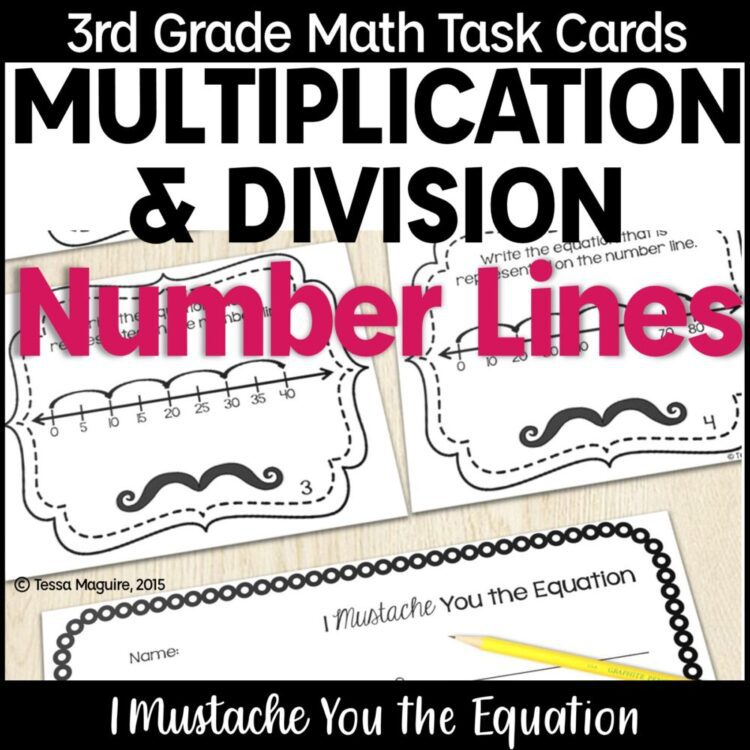I have other sets of similar task cards that I use throughout my multiplication and division units.  Part of why I like many of these is that I use the same task cards when practicing both- the directions just change.  Because many of them are visual images of the skill, they’re open ended and can be used to match whatever part of the unit you’re at.  You can see the entire Multiplication & Division Task Cards Bundle by clicking the image below.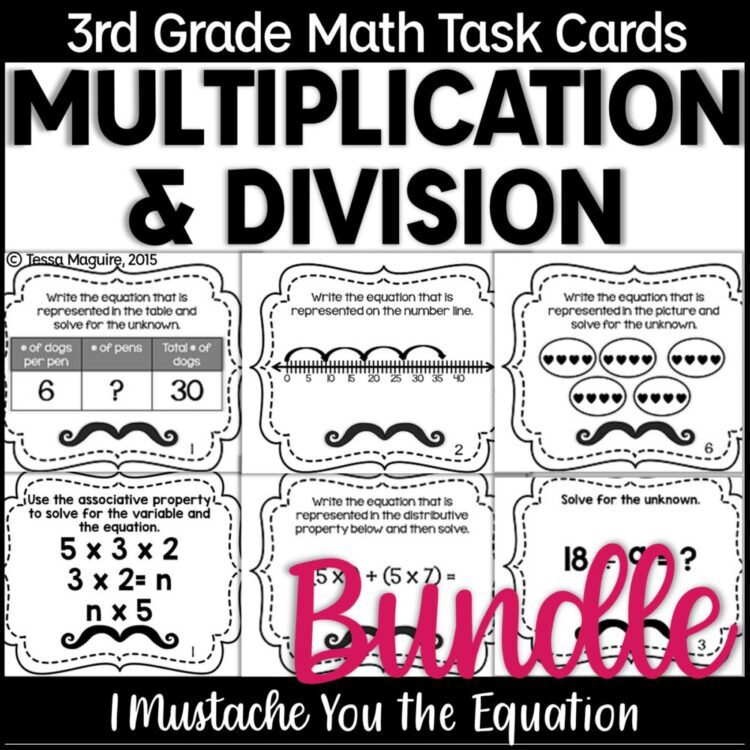## Spider Math- Hands-On Multiplication Unit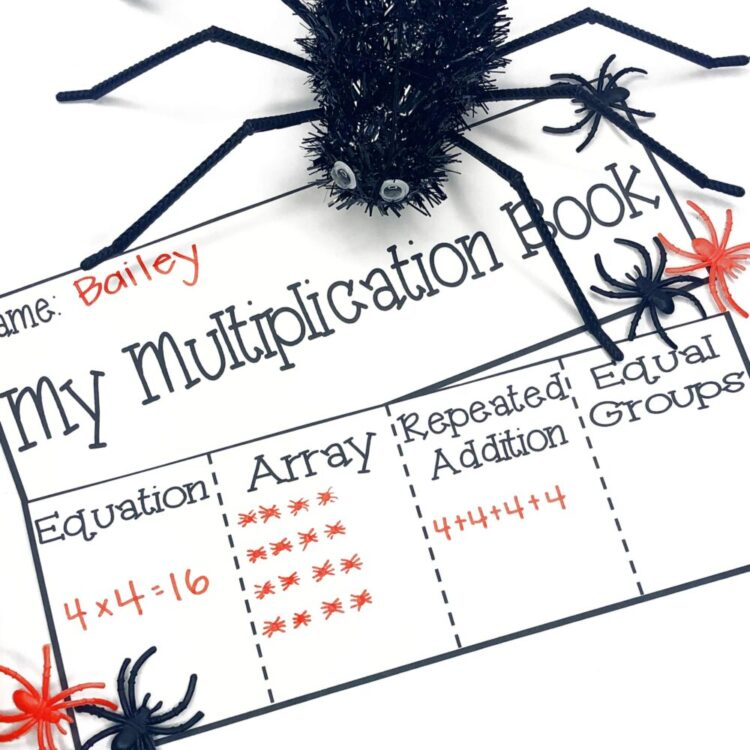If we’re doing our Multiplication and Division unit in late September and October, I use my Spider Math unit to reinforce our work using spider rings as manipulatives.  Students use the rings to make arrays and equal groups as they solve equations.  I bought the rings from Wal-Mart on clearance several years ago and keep them year after year.  This unit is perfect around Halloween.  I’ve also used this somewhat thematically as we studied life cycles of spiders and read Charlotte’s Web.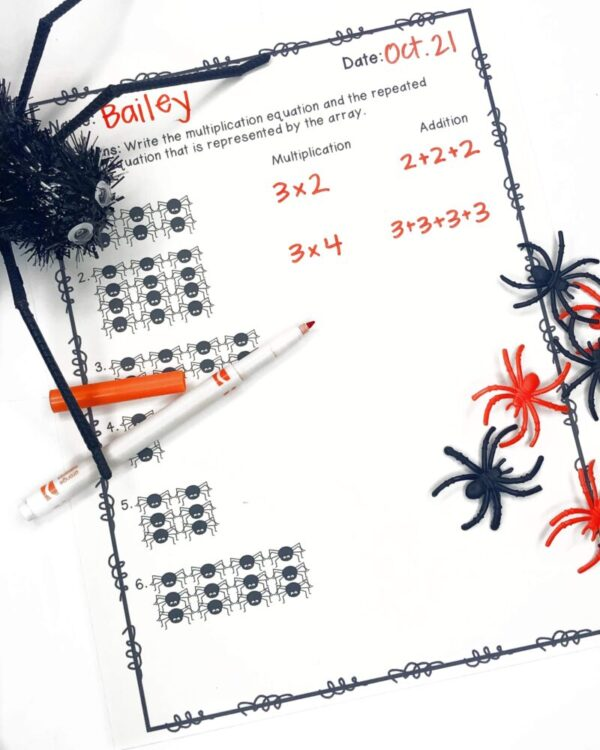My Spider Math unit includes multiplication vocabulary posters, a craftivity, games, and printables for throughout your multiplication unit.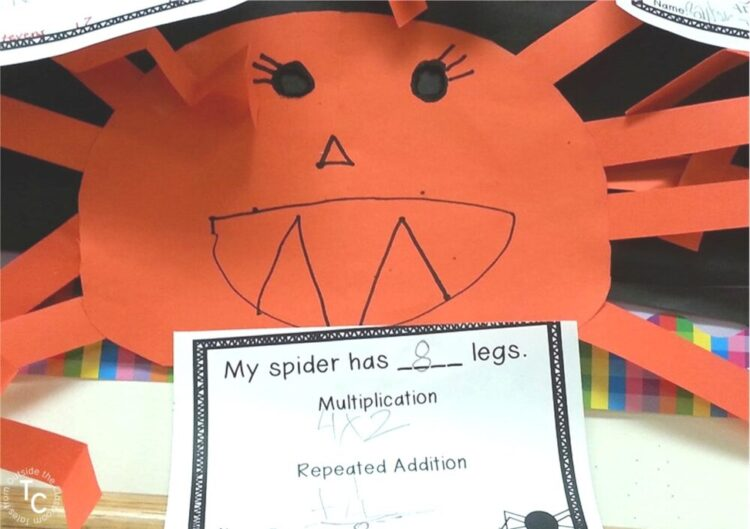These are the strategies resources I use while introducing multiplication with my students.  You can see how I introduce division , introduce the distributive property, and build multiplication fact fluency.  You can also see more about how I teach my math lessons in my small groups by reading my Math Block post.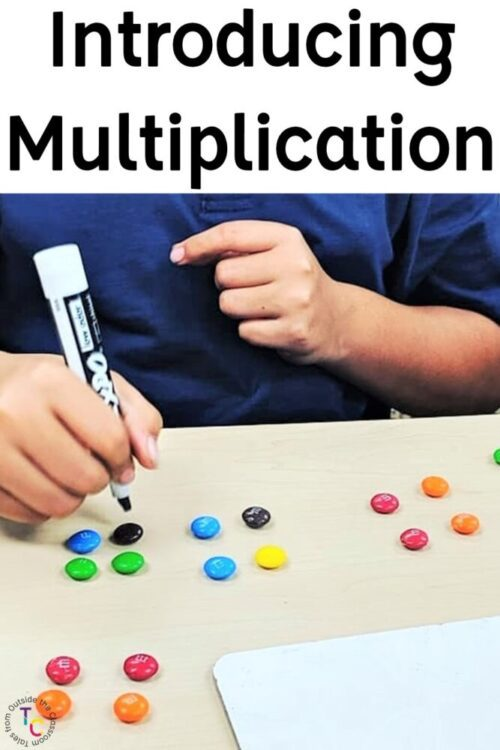This site uses Akismet to reduce spam. Learn how your comment data is processed.

## Hi! I'm Tessa!I’ve spent the last 15 years teaching in 1st, 2nd, and 3rd grades, and working beside elementary classrooms as an instructional coach and resource support. I’m passionate about math, literacy, and finding ways to make teachers’ days easier. I share from my experiences both in and out of the elementary classroom.

### ▹ SEARCH ◃